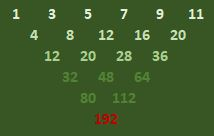# Problem 50073. Number Puzzles - 021

Borrowing the idea from Pascal Triangle, the following operation can be performed. Starting with an array of the first N odd numbers (where N is greater than 1), the following upside-down triangle of numbers can be formed where starting from the second row, the number is determined by adding the two neighboring numbers in the previous row.Given N, determine the last number at the bottom of the triangle (i.e., the red number in the illustration above).

### Solution Stats

88.89% Correct | 11.11% Incorrect
Last Solution submitted on Jun 15, 2023

### Community Treasure Hunt

Find the treasures in MATLAB Central and discover how the community can help you!

Start Hunting!